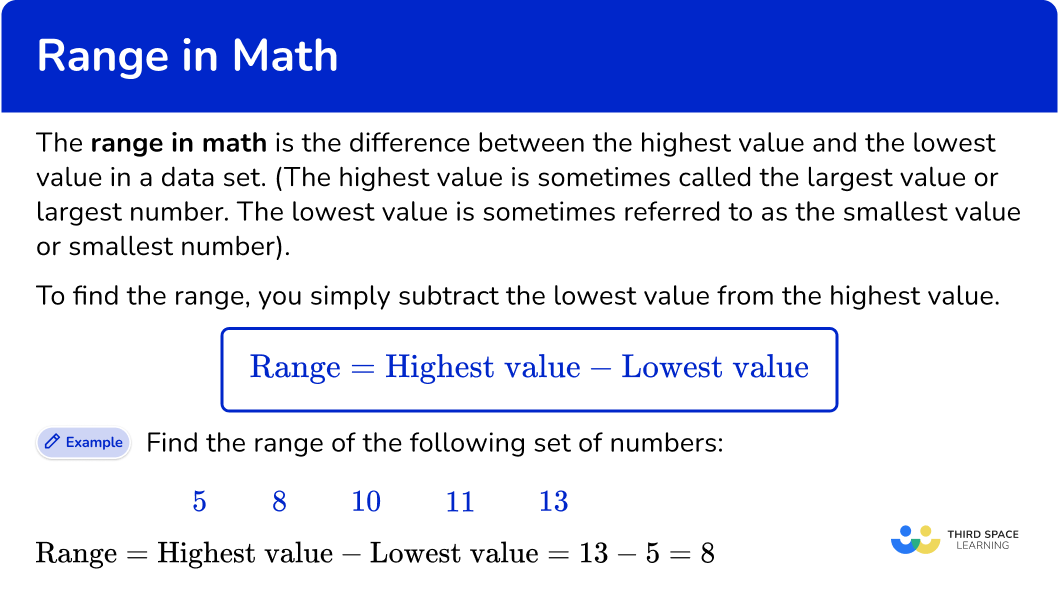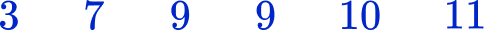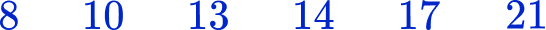Math resources Statistics and probability

Averages and range

Range in math

# Range in math

Here you will learn about the range in math, including what the range is and how to calculate it. You will also learn how to solve problems involving the range.

Students will first learn about range in math as part of statistics and probability in 6 th grade.

## What is range in math?

The range in math is the difference between the highest value and the lowest value in a data set. (The highest value is sometimes called the largest value, largest number, or maximum value.

The lowest value is sometimes referred to as the smallest value, smallest number, or minimum value).

To find the range, you simply subtract the lowest value from the highest value.

\text{Range}=\text{highest value}-\text{lowest value}

For example, find the range of the following set of numbers:

5 \hspace{0.6cm} 8 \hspace{0.6cm} 10 \hspace{0.6cm} 11 \hspace{0.6cm} 13

\text{Range}=\text{highest value}-\text{lowest value}=13-5=8

### What is range in math?## Common Core State Standards

How does this relate to 6 th grade math?

• Grade 6 – Statistics and Probability (6.SP.A.2)
Understand that a set of data collected to answer a statistical question has a distribution which can be described by its center, spread, and overall shape.

## How to calculate range

In order to calculate the range:

1. Identify the highest value.
2. Identify the lowest value.
3. Subtract the lowest value from the highest value.

## Range in math examples

### Example 1: finding the range for an ordered list of numbers

Find the range of this set of numbers.

1. Identify the highest value.

Look through the set of values and find the highest value (or highest number).

The highest value is 15.

2Identify the lowest value.

Look through the set of values and find the lowest value (or lowest number).

The lowest value is 3.

3Subtract the lowest value from the highest value.

The range is the difference between the highest and lowest values.

\text{Range}=\text{highest value}-\text{lowest value}=15-3=12

The range is 12.

### Example 2: finding the range for an ordered list of numbers

Find the range of this set of numbers

Look through the set of values and find the highest value (or highest number).

The highest value is 18.

Look through the set of values and find the lowest value (or lowest number).

The lowest value is 1.

The range is the difference between the highest and lowest values.

\text{Range}=\text{highest value}-\text{lowest value}=18-1=17

The range is 17.

## How to solve problems involving range

In order to use the range with problem solving:

1. Write down how to find the range.
2. Substitute the values you are given.
3. Calculate the missing value.

## Problems involving range examples

### Example 3: finding the highest value

The range of a set of data is 12.

The lowest value is 25.

Find the highest value.

\text{Range}=\text{highest value}-\text{lowest value}

25=\text{highest value}-12

To find the highest value you will need to add the range to the lowest value.

\text{Highest value}=12+25=37

The highest value is 37.

This answer can be doubled checked:

\text{Range}=\text{highest value}-\text{lowest value}=37-12=25

### Example 4: finding the highest value

The range of a set of data is 14.

The lowest value is 38.

Find the highest value.

\text{Range}=\text{highest value}-\text{lowest value}

14=\text{highest value}-38

To find the highest value you will need to add the range to the lowest value.

\text{Highest value}=38+14=52

The highest value is 52.

This answer can be doubled checked:

\text{Range}=\text{highest value}-\text{lowest value}=52-38=14

### Example 5: finding the lowest value

The range of a set of data is 8.

The highest value is 14.

Find the lowest value.

\text{Range}=\text{highest value}-\text{lowest value}

8=14-\text{lowest value}

To find the lowest value you will need to subtract the range from the highest value.

\text{lowest value}=14-8=6

The lowest value is 6.

This answer can be doubled checked:

\text{Range}=\text{highest value}-\text{lowest value}=14-6=8

### Example 6: finding the lowest number

The range of a set of data is 17.

The highest value is 28.

Find the lowest value.

\text{Range}=\text{highest value}-\text{lowest value}

17=28-\text{lowest value}

To find the lowest value you will need to subtract the range from the highest value.

\text{Lowest value}=28-17=11

The lowest value is 11.

This answer can be doubled checked:

\text{Range}=\text{highest value}-\text{lowest value}=28-11=17

### Teaching tips for range in math

• It is helpful to place all data points in ascending order first. This will make it easier for students to identify the highest value and the lowest value.

• Give students data sets containing various types of data points, including fractions, decimals, and whole numbers. Eventually increase the complexity of their practice problems by including negative numbers and positive numbers within a data set.

• Provide worksheets that include word problems or scenarios where students have to interpret the data and determine the range in a real-life context. This encourages critical thinking and helps students see the relevance of the concept.

### Easy mistakes to make

• Using the incorrect values
Sometimes the list of values is not in order of size. Make sure that you are definitely using the lowest and highest values. It can help to put the data set in ascending order first before determining the lowest value and highest value.

• Not calculating the range
Writing the range as lowest number to highest number with a dash in between is incorrect. To calculate the range, you need to find the difference between these values.
For example, what is the range of this set of numbers?
1 \hspace{0.6cm} 3 \hspace{0.6cm} 6 \hspace{0.6cm} 8
The range is NOT 1-8 or 1- to -8
To find the range, you subtract the lowest value from the highest value:
8-1=7
So the range is 7.

• Confusing range for mean, median, or mode
Students hear the terms mean, median, mode, and range together often and may confuse them. Mean is the average value of a data set with numerical values, median is the middle number or middle value when the list of values is placed in ascending order, mode is the piece of data with the highest frequency within the data set, and range is the difference between the highest value and the lowest value in the data set.

### Practice range in maths questions

1. Find the range of this data set:141398The range is calculated by finding the difference between the highest value and the lowest value.

\text{Range}=\text{highest value}-\text{lowest value}=11-3=8

2. Find the range of this data set:29141328The range is calculated by finding the difference between the highest and lowest values.

\text{Range}=\text{highest value}-\text{lowest value}=21-8=13

3. The range of a set of data is 6. The lowest value is 5. What is the highest value?

10111213The highest value is found by adding the range on to the lowest value.

\text{Highest value}=\text{lowest value}+\text{Range}=5+6=11

4. The range of a set of data is 17. The lowest value is 14. What is the highest value?

343031The highest value is found by adding the range on to the lowest value.

\text{Highest value}=\text{lowest value}+\text{Range}=14+17=31

5. The range of a set of data is 9. The highest value is 17. What is the lowest value?

782627The highest value is found by subtracting the range from the highest value.

\text{Lowest value}=\text{highest value}-\text{Range}=17-9=8

6. The range of a set of data representing the test scores of a high school math class is 26. The highest value is 98. What is the lowest value?

267212474The highest value is found by subtracting the range from the highest value.

\text{Lowest value}=\text{highest value}-\text{Range}=98-26=72

## Range in math FAQs

What is the range in math?

The range in math is the difference between the highest value and the lowest value in a data set.

How do you find the range in math?

To find the range, you simply subtract the lowest value of the data set from the highest value.

How is the range of a data set affected by outliers?

Outliers can significantly affect the range of a data set. If there are extreme values that are much larger or smaller than the rest of the data, the range will be skewed or stretched to include these outlier values. This means that the presence of outliers can result in a larger range if there is a high outlier or a low outlier.

What are descriptive statistics?

Descriptive statistics are methods used to summarize or organize a data set. Measures of central tendency such as the mean, median, mode, and range are common descriptive statistics that give a quick overview of the data set.

## The next lessons are

• Frequency table
• Cumulative frequency
• Types of data
• Representing data
• Units of measurement

## Still stuck?

At Third Space Learning, we specialize in helping teachers and school leaders to provide personalized math support for more of their students through high-quality, online one-on-one math tutoring delivered by subject experts.

Each week, our tutors support thousands of students who are at risk of not meeting their grade-level expectations, and help accelerate their progress and boost their confidence.